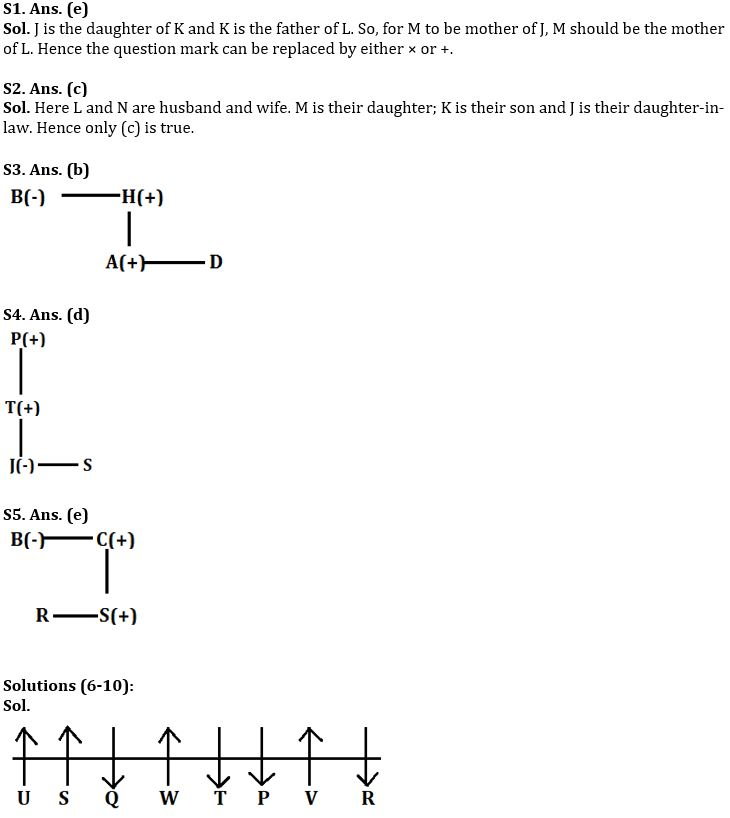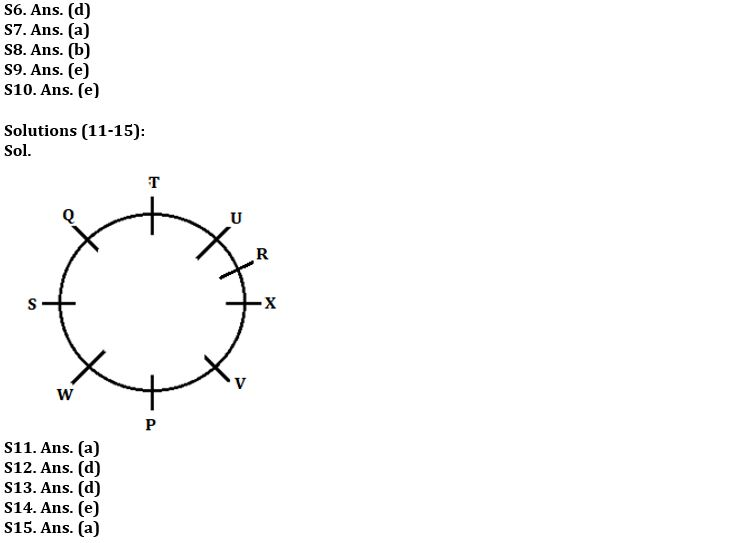Latest Banking jobs   »

# Reasoning Ability Quiz For LIC AAO 2023- 24th January

Directions (1-2): Read the following information carefully and answer the question which follows:
IF ‘A × B’ means ‘A is son of B’
IF ‘A + B’ means ‘A is daughter of B’
IF ‘A ÷ B’ means ‘A is wife of B’
IF ‘A – B’ means ‘A is Father of B’

Q1. What will come in the place of the question mark, to establish that M is mother of J in the expression?
‘J + K – L ? M’
(a) +
(b) ×
(c) –
(d) ÷
(e) Either (a) or (b)

Q2. Which of the following relations are true based upon the relations given in the question?
J ÷ K × L – M + N’?
(a) K is brother of N
(b) J is daughter-in-law of M
(c) J is daughter-in-law of N
(d) N is daughter of L
(e) None is true

Directions (3-5): Study the following information carefully and answer the questions which follow–
‘P – Q’ means ‘P is father of Q’
‘P ÷ Q’ means ‘P is sister of Q’
‘P × Q’ means ‘P is mother of Q’
‘P + Q’ means ‘P is brother of Q’

Q3. Which of the following means ‘A is nephew of B’?
(a) A + C – B × K
(b) B ÷ H – A + D
(c) B ÷ G – A ÷ R
(d) B + T × A ÷ E
(e) None of these

Q4. Which of the following means ‘P is grandfather of J’?
(a) J ÷ W – U – P
(b) P × G + J ÷ A
(c) P – B ÷ J ÷ R
(d) P – T – J ÷ S
(e) None of these

Q5. How is R related to B in the expression ‘B ÷ C – S + R’?
(a) aunt
(b) niece
(c) nephew
(d) None of these
(e) Cannot be determined

Directions (6-10): Study the information and answer the given questions:
Eight persons P, Q, R, S, T, U, V and W are sitting in row. Some of them facing north and some of them facing south but not necessary in same order. Not more than two adjacent persons face same direction.
V faces north. T sits third to the right of R who sits one of the extreme ends. S sits third to the right of T. P and Q faces same direction. The persons who are sitting at extreme ends face opposite direction to each other’s. Q sits immediate left of W. One person sit between P and W. P sits left of T. U sits left of S. U is not immediate neighbor of R. V face opposite direction to Q.

Q6. Who among the following person sits immediate left of V?
(a) R
(b) S
(c) T
(d) P
(e) W

Q7. How many persons sit between W and V?
(a) Two
(b) One
(c) None
(d) Three
(e) Four

Q8. Four of the following five are alike in certain way based from a group, find the one that does not belong to that group?
(a) Q
(b) V
(c) P
(d) T
(e) R

Q9. How many persons face south direction?
(a) Two
(b) One
(c) None
(d) Three
(e) Four

Q10. Who among the following person sits 2nd to the left of Q?
(a) W
(b) P
(c) U
(d) R
(e) T

Directions (11-15): Study the information carefully and answer the questions given below.
Nine persons P, Q, R, S, T, U, V, W and X are sitting in a circle but not necessary in same order. All of them face inside.
R sits third to the right of P. Two persons sit between R and Q. U sits second to the right of X who is not immediate neighbor of P. Two persons sit between T and W. S is third to the left of V. S is not immediate neighbor of T and P who does not sit immediate right of V.

Q11. How many persons are sitting between S and P when counted from left of P?
(a) One
(b) Two
(c) Four
(d) Three
(e) None

Q12. Who among the following sits 4th to right of W?
(a) V
(b) P
(c) S
(d) R
(e) None of these

Q13. How many persons sit between Q and X when counted right of Q?
(a) Two
(b) One
(c) Three
(d) More than three
(e) None

Q14. Who sits second to the right of U?
(a) W
(b) T
(c) V
(d) P
(e) None of these

Q15. If W and T interchange their positions, then who sits second to the right of W ?
(a) S
(b) P
(c) R
(d) T
(e) None of these

Solutions## FAQs

### When will the LIC AAO Prelims exam be held?

The LIC AAO Prelims exam will be held on 17th & 20th of February 2023 (tentatively).

#### Congratulations!